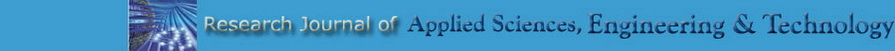It is the cache of http://maxwellsci.com/jp/abstract.php?jid=RJASET&no=266&abs=46. It is a snapshot of the page. The current page could have changed in the meantime.
Tip: To quickly find your search term on this page, press Ctrl+F or ⌘-F (Mac) and use the find bar.

Maxwell Science/Journal PageAbstract
 2013 (Vol. 5, Issue: 06)
 Article Information: Nonlinear Forced Vibration Analysis for Thin Rectangular Plate on Nonlinear Elastic Foundation Zhong Zhengqiang, Xiao Yonggang and Yang Cuiping Corresponding Author:  Zhong Zhengqiang  Key words:  Amplitude-frequency characteristic, forced vibration, nonlinear elastic foundation, thin rectangular plate, , , Vol. 5 , (06): 2163-2167
 Submitted Accepted Published July 31, 2012 September 03, 2012 February 21, 2013
 Abstract:
 Nonlinear forced vibration is analyzed for thin rectangular plate with four free edges on nonlinear elastic foundation. Based on Hamilton variation principle, equations of nonlinear vibration motion for thin rectangular plate under harmonic loads on nonlinear elastic foundation are established. In the case of four free edges, viable expressions of trial functions for this specification are proposed, satisfying all boundary conditions. Then, equations are transformed to a system of nonlinear algebraic equations by using Galerkin method and are solved by using harmonic balance method. In the analysis of numerical computations, the effect on the amplitude-frequency characteristic curve due to change of the structural parameters of plate, parameters of foundation and parameters of excitation force are discussed.
 Abstract PDF HTML
Cite this Reference:
 Zhong Zhengqiang, Xiao Yonggang and Yang Cuiping, 2013. Nonlinear Forced Vibration Analysis for Thin Rectangular Plate on Nonlinear Elastic Foundation.  Research Journal of Applied Sciences, Engineering and Technology, 5(06): 2163-2167.ISSN (Online):  2040-7467 ISSN (Print):   2040-7459